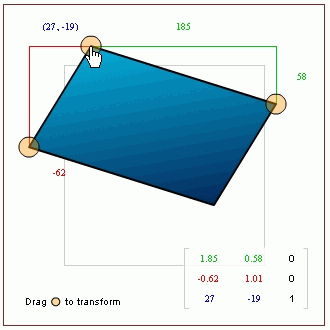Search IntMath
Close

450+ Math Lessons written by Math Professors and Teachers

5 Million+ Students Helped Each Year

1200+ Articles Written by Math Educators and Enthusiasts

Simplifying and Teaching Math for Over 23 Years

# Matrices and Flash games

By Murray Bourne, 18 Nov 2007

Matrices are useful whenever we need to transform one geometric shape into something else.

This excellent tutorial from senocular.com has some good example Flash files showing how the Flash matrix transform matrix works:

Understanding the Transformation Matrix in Flash 8

The tutorial includes matrix multiplication and the inverse of a matrix and has some applications at the end. Here's a screen shot from the tutorial:Matrices are also useful for telling robots what to do (the transformations in this case are in 3D).

### 2 Comments on “Matrices and Flash games”

1. Krishna Kumar says:

How to embed these games in PHP website? I want to embed in wordpress site.

2. Murray says:

@Krishna: Nobody is doing development in Flash any more, since it does not work on mobile devices. it would be better to use something like JSXGraph.

### Comment Preview

HTML: You can use simple tags like <b>, <a href="...">, etc.

To enter math, you can can either:

1. Use simple calculator-like input in the following format (surround your math in backticks, or qq on tablet or phone):
a^2 = sqrt(b^2 + c^2)
(See more on ASCIIMath syntax); or
2. Use simple LaTeX in the following format. Surround your math with $$ and $$.
$$\int g dx = \sqrt{\frac{a}{b}}$$
(This is standard simple LaTeX.)

NOTE: You can mix both types of math entry in your comment.